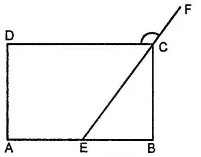Consider the following parallelograms. Find the values of the unknowns x, y, z.Asked by Aaryan | 1 year ago |  119

##### Solution :-

(i) x + 100° = 180° (Adjacent angles are supplementary)

x = 80°

z = x = 80º(Opposite angles are equal)

y = 100° (Opposite angles are equal)

(ii) 50° + y = 180° (Adjacent angles are supplementary)

y = 130°

x = y = 130° (Opposite angles are equal)

z = x = 130º (Corresponding angles)

(iii) x = 90° (Vertically opposite angles)

x + y + 30° = 180° (Angle sum property of triangles)

120° + y = 180°

y = 60°

z = y = 60° (Alternate interior angles)

(iv) z = 80° (Corresponding angles)

y = 80° (Opposite angles are equal)

x+ y = 180° (Adjacent angles are supplementary)

x = 180° - 80° = 100°

(v) y = 112° (Opposite angles are equal)

x+ y + 40° = 180° (Angle sum property of triangles)

x + 112° + 40° = 180°

x + 152° = 180°

x = 28°

z = x = 28° (Alternate interior angles)

Answered by Sakshi | 1 year ago

### Related Questions

#### In the given figure, PQRS is a kite. Find the values of x and y.

In the given figure, PQRS is a kite. Find the values of x and y.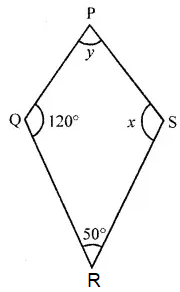#### In the given isosceles trapezium ABCD, ∠C = 102°. Find all the remaining angles of the trapezium.

In the given isosceles trapezium ABCD, ∠C = 102°. Find all the remaining angles of the trapezium.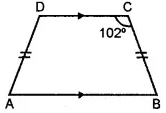#### In the given figure, ABCD is a rhombus and ∠ABD = 50°. Find :

In the given figure, ABCD is a rhombus and ∠ABD = 50°. Find :

(i) ∠CAB

(ii) ∠BCD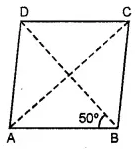#### In the given figure, ABCD is a rectangle and diagonals intersect at O. If ∠AOB = 118°, find

In the given figure, ABCD is a rectangle and diagonals intersect at O. If ∠AOB = 118°, find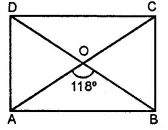(i) ∠ABO

(iii) ∠OCB